# How to convert a rational number to a decimal?

• Last Updated : 24 May, 2022

A number System can be defined as a system of writing to express numbers. It represents numbers in mathematical notation using a set of digits and symbols in a consistent manner. There are different types of numbers included in the number system like real numbers, whole numbers, integers, even, odd, prime numbers, rational, irrational, etc. The number system provides a unique representation for each number. There are different types of number systems available in Mathematics. They are- Decimal number system, Binary number system, Octal number system, and Hexadecimal number system.

The value of any digit in a number can be determined mainly by three measures. Those are,

• The digit.
• Position of a digit in the number (Like 10’s place 100’s place)
• Base of the number system.

### What is a Rational Number?

A rational number is a number that can be expressed as the ratio of two integers i.e. in fraction form P/Q and the denominator in a rational number should not be equal to zero.

A few examples of rational numbers are 1/2, 22/7, 13/3, 7/12, etc.

### What is a Decimal Number?

A decimal number is a number that is used in representing integer and non-integer numbers. A decimal number has a whole number and the fractional part separated by a dot. The dot present between the whole number and the fractional part is called the decimal point.

### How do you convert a rational number to a decimal?

We divide the numerator by the denominator to convert a rational number to a decimal. To convert a rational number to a decimal, we simply convert it to a fraction. The numerator is then divided by the denominator, yielding the division’s exact value.

Here are the steps to convert fractions to decimals is as follows:

1. Make the fraction an incorrect fraction if it’s a mixed number.
2. Subtract the denominator from the numerator.
3. Round the decimal off if the division does not come out evenly.

Let’s look into a few sample problems on how to convert a rational number to a decimal number using the Division method.

### Sample Problems

Problem 1: Convert a rational number 3/2 into a decimal number.

Solution: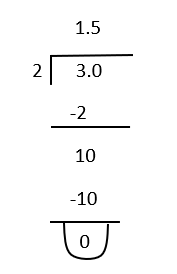The decimal form of number 3/2 is 1.5

Problem 2: Convert a rational number 5/6 into a decimal number.

Solution: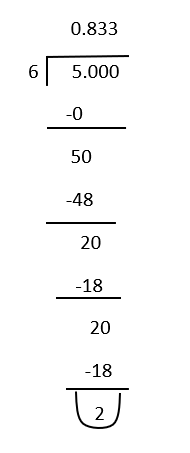Even after performing further division we get 2 as remainder. So the result we get is repeating decimal.

The decimal form of number 5/6 is 0.8333…

Problem 3: Convert a rational number 1/3 into a decimal number.

Solution: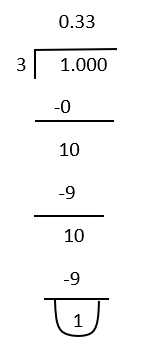Even after performing further division we get 1 as remainder. So the result we get is repeating decimal.

The decimal form of number 1/3 is 0.333…

Problem 4: Convert the number 12/0 into decimal form.

Solution:

First of all the number 12/0 is not rational because the denominator should not be zero in rational number. Even here we can’t perform division operation between 12 and 0 because any number cannot be divided by zero.

Problem 5: Convert the rational number 22/7 into decimal form.

Solution: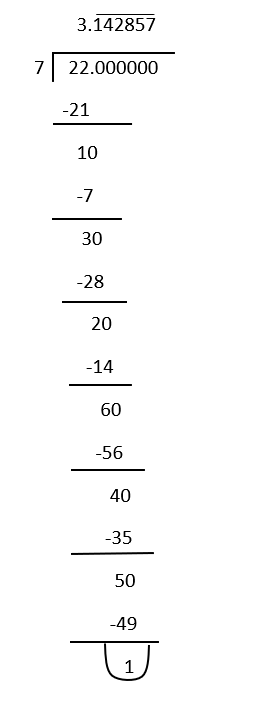Here the decimal part of 142857 will repeat again and again on further simplification.

So the decimal form of 22/7 is 3.\bar{142857}.

Problem 6: Convert the rational number 25/4 into decimal form.

Solution: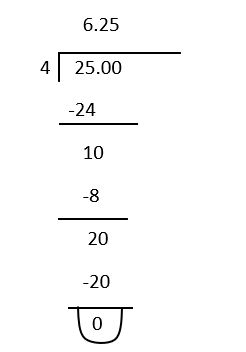Here we got a non repeating decimal form for the given rational number.

So decimal form of a rational number 25/4 is 6.25.

My Personal Notes arrow_drop_up
Recommended Articles
Page :